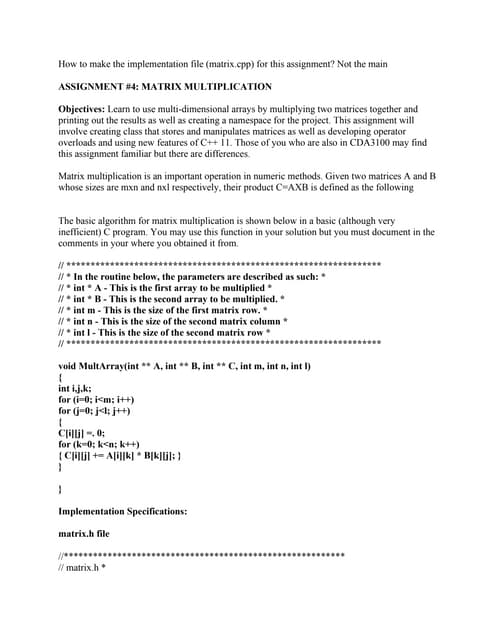Anúncio

# For assignment submission- use the format listed in the syllabus- Use.docx

8 de Feb de 2023Próximos SlideSharesHow to make the implementation file (matrix-cpp) for this assignment-.pdf
Carregando em ... 3
1 de 2
Anúncio

### For assignment submission- use the format listed in the syllabus- Use.docx

1. For assignment submission, use the format listed in the syllabus. Use comments in your code (5 points will be deducted from each problem missing comments) Your solution must include header, implementation file, and client files. Consider a graphics system that has classes for various figures rectangles, squares, triangles, circles, and so on. For example, a rectangle might have data members for height, width, and center point, while a square and circle might have only a center point and an edge length or radius, respectively. In a well-designed system, these would be derived from a common class, Figure. You arc to implement such a system. The class Figure is the base class. You should add only Rectangle and Triangle classes derived from Figure. Each class has stubs for member tune lions erase and draw. Each of these member functions Outputs a message telling what function has been called and what the class of the calling object is. Since these are just stubs, they do nothing more than output this message. The member function center calls the erase and draw functions to erase and redraw the figure at the center. Since you have only stubs for erase and draw, center will not do any "centering" but will call the member functions erase and draw. Also add an output message in the member function center that announces that center is being called. The member functions should take no arguments. There are three parts to this project: Write the class definitions using no virtual functions. Compile and test. Make the base class member functions virtual. Compile and test. Explain the difference in results. Use the following main function for all testing: Solution class Polygon { public: virtual void draw() { cout<<"Base class draw"; } virtual void center() { cout<<"Base class center"; } }; class Rectangle: public Polygon { public: void draw() { cout<<"Rectangle class draw"; } void center() { cout<<"Rectangle class center"; } };
2. class Triangle: public Polygon { public: void draw() { cout<<"Triangle class draw"; } void center() { cout<<"Triangle class center"; } };
Anúncio